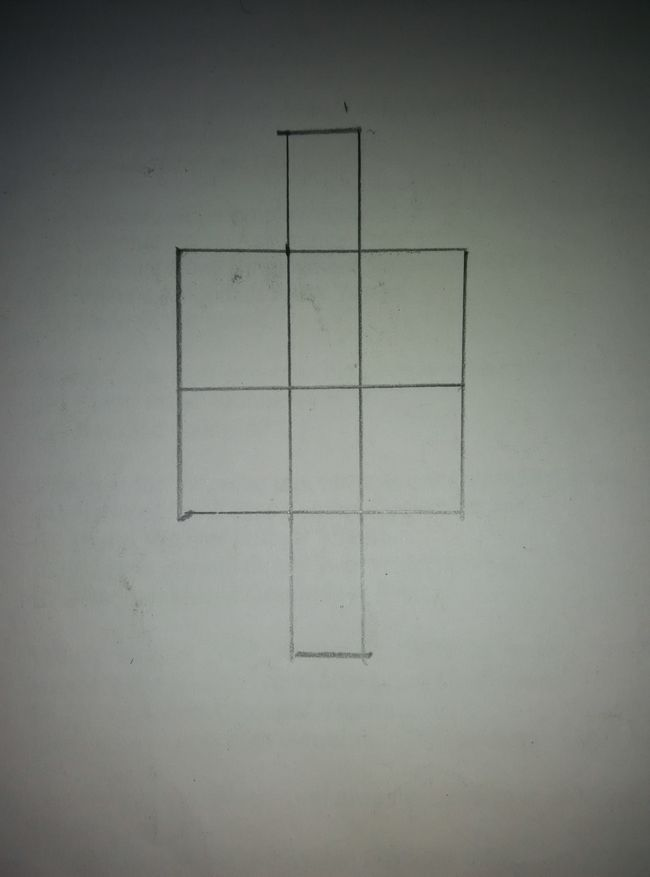# Rough figure

Probability Level 4Six P's have to be placed in the squares of the diagram given above such that each column contains at least one P.In how many ways can this be done?

×